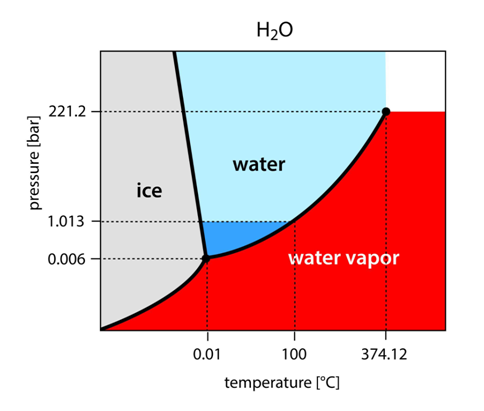top of page
• BWB Technologies

# Can I use a phase diagram to determine states of matter?

When it comes to physics, a state of matter is one of the distinct forms in which matter exists.

It’s understandable that you automatically think of the ‘big three’, which are solids, gasses and liquids.

That may also extend to plasmas, or maybe even Bose-Einstein condensates.

The latter is a state of matter which is usually formed when a gas of bosons (particles) at low densities is cooled to temperatures close to absolute zero.

Phases like these are better-suited to describing what state of matter is present in a system than any single definition.

## Identifying the phases of matter

Imagine a single ice cube in a closed system, where no heat light or energy is escaping.

Yes, the ice is solid and, yes, there is gaseous water vapour and liquid water present in the system.

With the application of phases to this problem, though, it gives scientists a statistical look at the overall system, with respect to the thermal energy and pressure of the system.

In this system of ice, there would be all three states of matter present.

However, the majority would be in the solid state, so the phase of the overall system would be known as being in the solid phase.

If this system had its temperature increased significantly, this could be totally different.

## Using a phase diagram to determine states of matter

A phase diagram is a chart used to show conditions at which thermodynamically distinct phases occur and coexist at equilibrium.

These diagrams are useful tools for scientists to investigate what state the majority of matter present in a system is at a given temperature and pressure.

There are a few important points on these graphs which are of note. The critical point is a temperature at which liquid and solid matter can no longer exist.

Meanwhile, the triple point is where all three standard states of matter exist at the same time.

Such points are used in a multitude of equations relating to states of matter and pressure temperature calculations for thermodynamics.

Below is a phase diagram for water as an example.## Can phase diagrams be used for other states of matter?

Phase diagrams can be increased to also include where plasma formation occurs, as well as where other states of matter occur.

However, they would be far further down the axis of temperature and pressure. This is due to the immense pressures and temperatures required to form plasmas and other states of matter.

Just think how much energy and pressure is present in the sun where these states occur.

bottom of page